### Home > A2C > Chapter Ch4 > Lesson 4.2.1 > Problem4-78

4-78.

Write a possible equation for each of these graphs. Assume that one mark on each axis is one unit. When you are in class, check your equations on a graphing calculator and compare your results with your teammates.

1.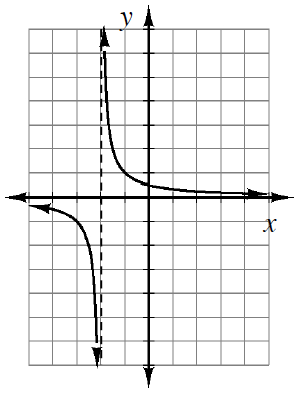Notice that this is a hyperbola $(y=\frac{1}{x})$.

1.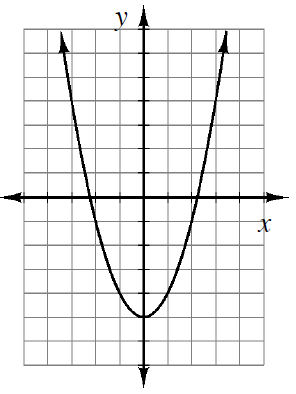Notice that the vertex is $(0,−5)$.

1.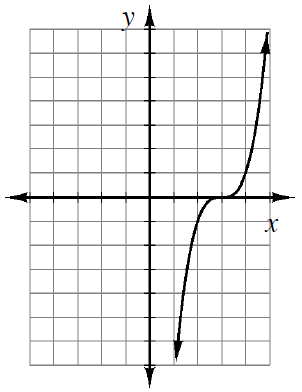Notice that this is a cubic graph.

1.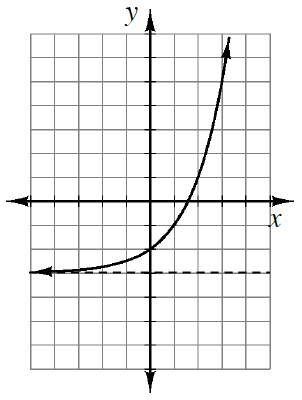Notice that this is an exponential graph.

1.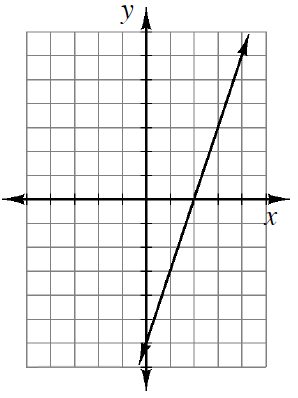Notice that the $y$-intercept is $(0,−6)$.

1.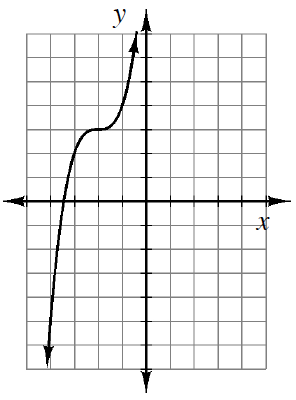See the hints from parts (a)-(e) to help you solve parts (f)-(i).

1.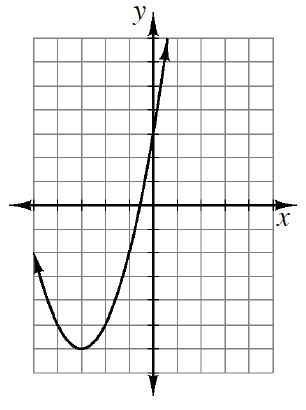1.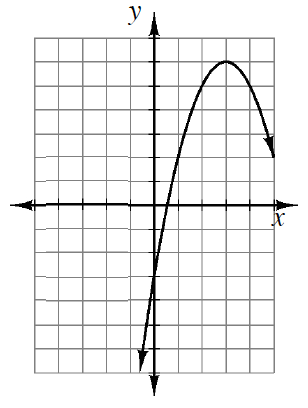1.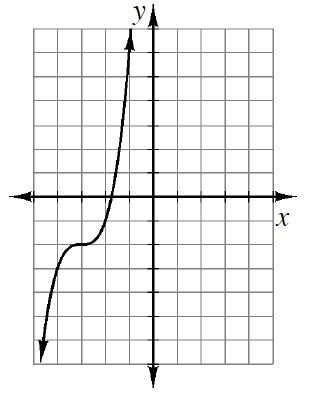Use the eTool below to graph the equations.
Click the link at right for the full version of the eTool: CCA2 2-95 HW eTool